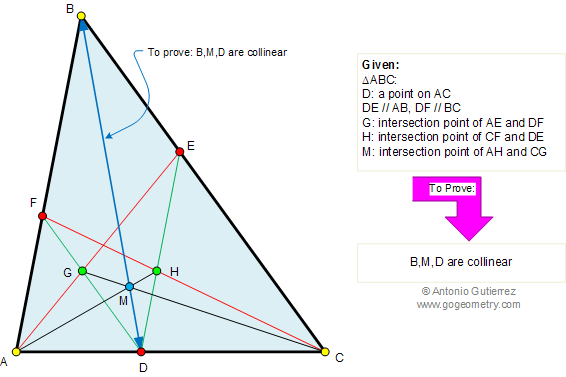Problem 390. Triangle, Parallel lines, Collinear points. Level: High School, SAT Prep, College geometry. The figure shows a triangle ABC with DE parallel to AB and DF parallel to BC. AE intersects DF at G, CF intersects DE at H, and AH intersects CG at M. Prove that the points B, M, and D are collinear.Recent Additions